# 1 Introduction

The aim of this vignette is to illustrate the use of pubh functions for common regression analysis in Public Health. In particular, the vignette shows the use of the following functions from pubh:

1. cosm_reg for reporting tables of coefficients.
2. cross_tbl for reporting tables of descriptive statistics by exposure of interest.
3. multiple for adjusting confidence intervals and p-values for post-hoc analysis.
4. get_r2 for accessing $$R^2$$ or pseudo-$$R^2$$ from regression models.
5. glm_coef for some special cases of regression models.

The advantages and limitations of glm_coef are:

1. Recognises the main models used in epidemiology/public health.
2. Automatically back-transforms estimates and confidence intervals, when the model requires it.
3. Can use robust standard errors for the calculation of confidence intervals.
• Standard errors are used by default.
• The use of standard errors is restricted by the following classes of objects (models): gee, glm and survreg.
4. Can display nice labels for the names of the parameters.
5. Returns a data frame that can be modified and/or exported as tables for publications (with further editing).

We start by loading relevant packages and setting the theme for the plots (as suggested in the Template of this package):

rm(list = ls())
library(tidyverse)
library(rstatix)
library(parameters)
library(performance)
library(jtools)
library(pubh)
library(sjlabelled)
library(sjPlot)

theme_set(sjPlot::theme_sjplot2(base_size = 10))
theme_update(legend.position = "top")
options('huxtable.knit_print_df' = FALSE)
options('huxtable.autoformat_number_format' = list(numeric = "%5.2f"))
knitr::opts_chunkset(comment = NA) # 2 Multiple Linear Regression For continuous outcomes there is no need of exponentiating the results unless the outcome was fitted in the log-scale. In our first example we want to estimate the effect of smoking and race on the birth weight of babies. We can generate factors and assign labels in the same pipe stream: data(birthwt, package = "MASS") birthwt <- birthwt %>% mutate( smoke = factor(smoke, labels = c("Non-smoker", "Smoker")), race = factor(race, labels = c("White", "African American", "Other")) ) %>% var_labels( bwt = 'Birth weight (g)', smoke = 'Smoking status', race = 'Race' ) Is good to start with some basic descriptive statistics, so we can compare the birth weight between groups. Graphical analysis: birthwt %>% box_plot(bwt ~ smoke, fill = ~ race)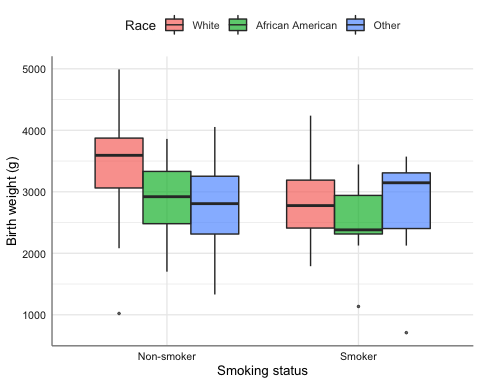Another way to compare the means between the groups is with gen_bst_df which estimates means with corresponding bootstrapped CIs. birthwt %>% gen_bst_df(bwt ~ race|smoke) %>% as_hux() %>% theme_pubh() Birth weight (g)LowerCIUpperCIRaceSmoking status 3428.753207.583619.51WhiteNon-smoker 2826.852664.992997.65WhiteSmoker 2854.502539.373139.82African AmericanNon-smoker 2504.002090.872851.15African AmericanSmoker 2815.782614.452994.04OtherNon-smoker 2757.172225.333138.67OtherSmoker We fit a linear model. model_norm <- lm(bwt ~ smoke + race, data = birthwt) Note: Model diagnostics are not be discussed in this vignette. Traditional output from the model: model_norm %>% Anova() Anova Table (Type II tests) Response: bwt Sum Sq Df F value Pr(>F) smoke 7322575 1 15.4588 0.0001191 *** race 8712354 2 9.1964 0.0001557 *** Residuals 87631356 185 --- Signif. codes: 0 '***' 0.001 '**' 0.01 '*' 0.05 '.' 0.1 ' ' 1 model_norm %>% parameters() Parameter | Coefficient | SE | 95% CI | t(185) | p ------------------------------------------------------------------------------------- (Intercept) | 3334.95 | 91.78 | [3153.89, 3516.01] | 36.34 | < .001 smoke [Smoker] | -428.73 | 109.04 | [-643.86, -213.60] | -3.93 | < .001 race [African American] | -450.36 | 153.12 | [-752.45, -148.27] | -2.94 | 0.004 race [Other] | -452.88 | 116.48 | [-682.67, -223.08] | -3.89 | < .001 model_norm %>% performance() # Indices of model performance AIC | BIC | R2 | R2 (adj.) | RMSE | Sigma ----------------------------------------------------------- 3012.223 | 3028.432 | 0.123 | 0.109 | 680.924 | 688.246 Table of coefficients for publication: model_norm %>% tbl_regression() %>% cosm_reg() %>% theme_pubh() %>% add_footnote(get_r2(model_norm), font_size = 9)  Variable Beta 95% CI p-value Smoking status <0.001 Non-smoker — — Smoker -429 -644, -214 Race <0.001 White — — African American -450 -752, -148 Other -453 -683, -223 R2 = 0.123 model_norm %>% glm_coef(labels = model_labels(model_norm)) %>% as_hux() %>% set_align(everywhere, 2:3, "right") %>% theme_pubh() %>% add_footnote(get_r2(model_norm), font_size = 9) ParameterCoefficientPr(>|t|) Constant3334.95 (3153.89, 3516.01)< 0.001 Smoking status: Smoker-428.73 (-643.86, -213.6)< 0.001 Race: African American-450.36 (-752.45, -148.27)0.004 Race: Other-452.88 (-682.67, -223.08)< 0.001 R2 = 0.123 Function glm_coef allows the use of robust standard errors. model_norm %>% glm_coef(se_rob = TRUE, labels = model_labels(model_norm)) %>% as_hux() %>% set_align(everywhere, 2:3, "right") %>% theme_pubh() %>% add_footnote(paste( get_r2(model_norm), "\n", "CIs and p-values estimated with robust standard errors."), font_size = 9) ParameterCoefficientPr(>|t|) Constant3334.95 (3144.36, 3525.53)< 0.001 Smoking status: Smoker-428.73 (-652.88, -204.58)< 0.001 Race: African American-450.36 (-734.09, -166.63)0.002 Race: Other-452.88 (-701.4, -204.35)< 0.001 R2 = 0.123 CIs and p-values estimated with robust standard errors. To construct the effect plot, we can use plot_model from the sjPlot package. The advantage of plot_model is that recognises labelled data and uses that information for annotating the plots. model_norm %>% plot_model("pred", terms = ~race|smoke, dot.size = 1.5, title = "")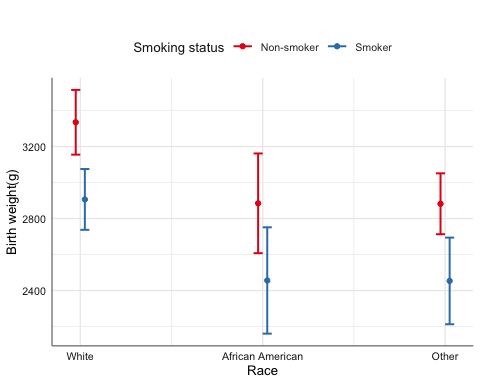When the explanatory variables are categorical, another option is emmip from the emmeans package. We can include CIs in emmip but as estimates are connected, the resulting plots look more messy, so I recommend emmip to look at the trace. emmip(model_norm, smoke ~ race) %>% gf_labs(y = get_label(birthwtbwt), x = "", col = "Smoking status")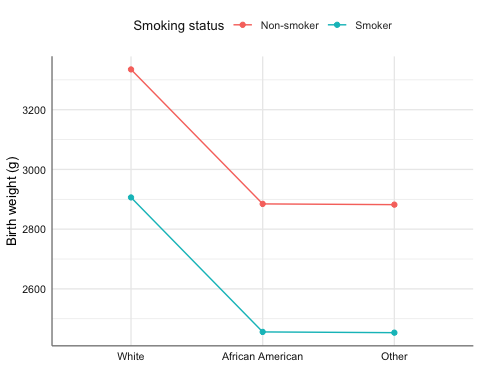# 3 Logistic Regression

For logistic regression we are interested in the odds ratios. We will look at the effect of amount of fibre intake on the development of coronary heart disease.

data(diet, package = "Epi")
diet <- diet %>%
mutate(
chd = factor(chd, labels = c("No CHD", "CHD"))
) %>%
var_labels(
chd = "Coronary Heart Disease",
fibre = "Fibre intake (10 g/day)"
)

diet %>% estat(~ fibre|chd) %>%
as_hux() %>% theme_pubh()
Coronary Heart DiseaseNMin.Max.MeanMedianSDCV
Fibre intake (10 g/day)No CHD288.00 0.60 5.35 1.75 1.69 0.58 0.33
CHD45.00 0.76 2.43 1.49 1.51 0.40 0.27
diet %>% na.omit() %>%
copy_labels(diet) %>%
box_plot(fibre ~ chd)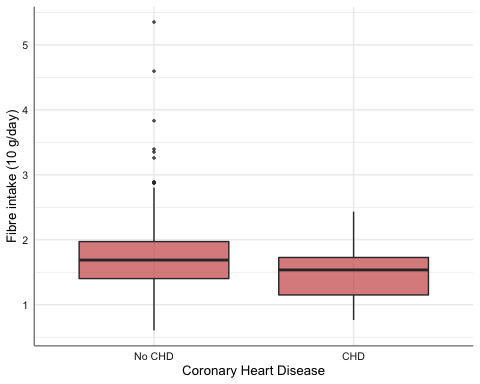We fit a linear logistic model:

model_binom <- glm(chd ~ fibre, data = diet, family = binomial)

Summary:

model_binom %>% parameters(exponentiate = TRUE)
Parameter   | Odds Ratio |   SE |       95% CI |     z |     p
--------------------------------------------------------------
(Intercept) |       0.95 | 0.58 | [0.30, 3.16] | -0.08 | 0.936
fibre       |       0.33 | 0.12 | [0.15, 0.66] | -2.94 | 0.003

Showing profiled confidence intervals.
model_binom %>% performance()
# Indices of model performance

AIC     |     BIC | Tjur's R2 |  RMSE | Sigma | Log_loss | Score_log | Score_spherical |   PCP
----------------------------------------------------------------------------------------------
257.535 | 265.151 |     0.028 | 0.337 | 0.875 |    0.381 |    -6.359 |           0.017 | 0.773

Table of coefficients for publication:

model_binom %>%
tbl_regression(exponentiate = TRUE) %>%
cosm_reg() %>% theme_pubh() %>%
add_footnote(get_r2(model_binom), font_size = 9)
 Variable OR 95% CI p-value Fibre intake (10 g/day) 0.33 0.15, 0.66 0.001 Tjur's R2 = 0.028

Effect plot:

model_binom %>%
plot_model("pred", terms = "fibre [all]", title = "")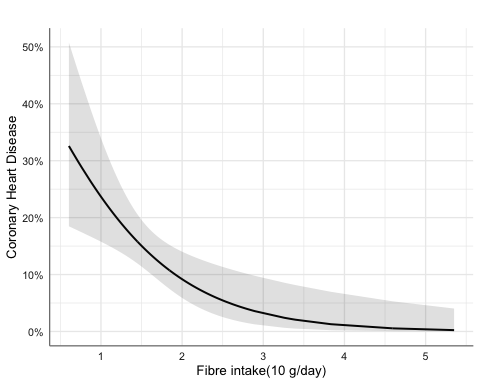## 3.1 Matched Case-Control Studies: Conditional Logistic Regression

We will look at a matched case-control study on the effect of oestrogen use and history of gall bladder disease on the development of endometrial cancer.

data(bdendo, package = "Epi")
bdendo <- bdendo %>%
mutate(
cancer = factor(d, labels = c('Control', 'Case')),
gall = factor(gall, labels = c("No GBD", "GBD")),
est = factor(est, labels = c("No oestrogen", "Oestrogen"))
) %>%
var_labels(
cancer = 'Endometrial cancer',
est = 'Oestrogen'
)

bdendo %>%
mutate(
cancer = relevel(cancer, ref = "Case"),
gall = relevel(gall, ref = "GBD"),
est = relevel(est, ref = "Oestrogen")
) %>%
copy_labels(bdendo) %>%
select(cancer, gall, est) %>%
tbl_strata(
strata = est,
.tbl_fun = ~ .x %>%
tbl_summary(by = gall)
) %>%
cosm_sum(bold = TRUE, head_label = " ") %>%
theme_pubh(2) %>%
set_align(1, everywhere, "center")
 Oestrogen No oestrogen GBD, N = 29 No GBD, N = 154 GBD, N = 12 No GBD, N = 120 Endometrial cancer Case 13 (45%) 43 (28%) 4 (33%) 3 (2.5%) Control 16 (55%) 111 (72%) 8 (67%) 117 (98%)
bdendo %>%
gf_percents(~ cancer|gall, fill = ~ est, position = "dodge", alpha = 0.6) %>%
gf_labs(
y = "Percent",
x = "",
fill = ""
)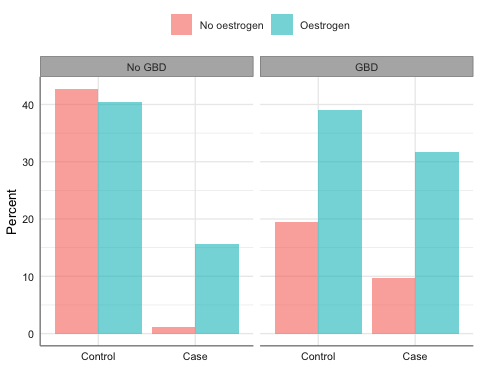We fit the conditional logistic model:

require(survival, quietly = TRUE)
model_clogit <- clogit(cancer == 'Case' ~ est * gall + strata(set), data = bdendo)
model_clogit %>%
glm_coef(labels = c("Oestrogen/No oestrogen", "GBD/No GBD",
"Oestrogen:GBD Interaction")) %>%
as_hux() %>% set_align(everywhere, 2:3, "right") %>%
theme_pubh() %>%
add_footnote(get_r2(model_clogit), font_size = 9)
ParameterOdds ratioPr(>|z|)
Oestrogen/No oestrogen14.88 (4.49, 49.36)< 0.001
GBD/No GBD18.07 (3.2, 102.01)0.001
Oestrogen:GBD Interaction0.13 (0.02, 0.9)0.039
Nagelkerke's R2 = 0.305

Creating data frame needed to construct the effect plot:

require(ggeffects, quietly = TRUE)
bdendo_pred <- ggemmeans(model_clogit, terms = c('gall', 'est'))

Effect plot:

bdendo_pred %>%
gf_pointrange(predicted + conf.low + conf.high ~ x|group, col = ~ x) %>%
levels(quine$Sex) <- c("Female", "Male") quine <- quine %>% var_labels( Days = "Number of absent days", Eth = "Ethnicity", Age = "Age group" ) Descriptive statistics: quine %>% cross_tbl(by = "Eth") %>% theme_pubh(2) %>% add_footnote("n (%); Median (IQR)", font_size = 9)  Ethnicity Aboriginal, N = 69 White, N = 77 Overall, N = 146 Sex Female 38 (55%) 42 (55%) 80 (55%) Male 31 (45%) 35 (45%) 66 (45%) Age group F0 13 (19%) 14 (18%) 27 (18%) F1 20 (29%) 26 (34%) 46 (32%) F2 20 (29%) 20 (26%) 40 (27%) F3 16 (23%) 17 (22%) 33 (23%) Lrn AL 40 (58%) 43 (56%) 83 (57%) SL 29 (42%) 34 (44%) 63 (43%) Number of absent days 15 (6, 32) 7 (4, 16) 11 (5, 23) n (%); Median (IQR) quine %>% box_plot(Days ~ Age|Sex, fill = ~ Eth)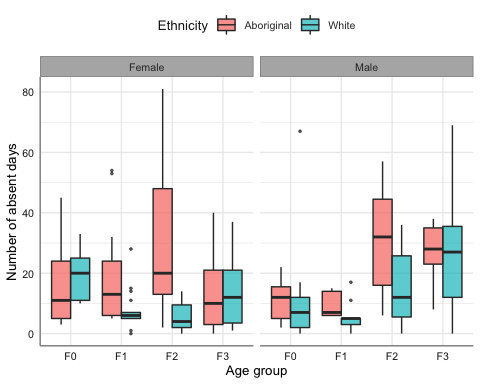We start by fitting a standard Poisson linear regression model: model_pois <- glm(Days ~ Eth + Sex + Age, family = poisson, data = quine) model_pois %>% tbl_regression(exponentiate = TRUE) %>% cosm_reg() %>% theme_pubh() %>% add_footnote(get_r2(model_pois), font_size = 9)  Variable IRR 95% CI p-value Ethnicity <0.001 Aboriginal — — White 0.59 0.54, 0.64 Sex 0.012 Female — — Male 1.11 1.02, 1.21 Age group <0.001 F0 — — F1 0.80 0.70, 0.91 F2 1.42 1.26, 1.60 F3 1.35 1.19, 1.53 Nagelkerke's R2 = 0.896 model_pois %>% performance() # Indices of model performance AIC | BIC | Nagelkerke's R2 | RMSE | Sigma | Score_log | Score_spherical ------------------------------------------------------------------------------------ 2342.982 | 2360.883 | 0.896 | 14.940 | 3.528 | -7.983 | 0.049 ## 4.1 Negative-binomial The assumption is that the mean is equal than the variance. If that is the case, deviance should be close to the degrees of freedom of the residuals. We can check for overdispersion: model_pois %>% check_overdispersion() # Overdispersion test dispersion ratio = 13.890 Pearson's Chi-Squared = 1944.553 p-value = < 0.001 Overdispersion detected. Thus, we have over-dispersion. One option is to use a negative binomial distribution. model_negbin <- MASS::glm.nb(Days ~ Eth + Sex + Age, data = quine) model_negbin %>% tbl_regression(exponentiate = TRUE) %>% cosm_reg() %>% theme_pubh() %>% add_footnote(get_r2(model_negbin), font_size = 9)  Variable IRR 95% CI p-value Ethnicity <0.001 Aboriginal — — White 0.57 0.41, 0.77 Sex 0.7 Female — — Male 1.07 0.77, 1.47 Age group 0.023 F0 — — F1 0.69 0.43, 1.09 F2 1.20 0.75, 1.89 F3 1.29 0.80, 2.06 Nagelkerke's R2 = 0.21 model_negbin %>% performance() # Indices of model performance AIC | BIC | Nagelkerke's R2 | RMSE | Sigma | Score_log | Score_spherical ------------------------------------------------------------------------------------ 1109.653 | 1130.538 | 0.210 | 15.087 | 1.095 | -3.908 | 0.067 Effect plot: model_negbin %>% plot_model(type = "pred", terms = c("Age", "Eth"), dot.size = 1.5, title = "")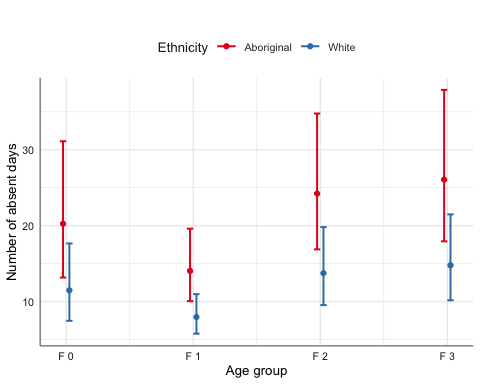emmip(model_negbin, Eth ~ Age|Sex) %>% gf_labs(y = "Number of absent days", x = "Age group", col = "Ethnicity")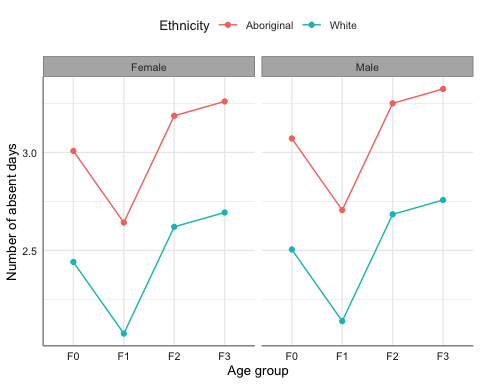## 4.2 Adjusting CIs and p-values for multiple comparisons We adjust for multiple comparisons: multiple(model_negbin, ~ Age|Eth)$df
   contrast        Eth ratio   SE null z.ratio p.value lower.CL upper.CL
1   F1 / F0 Aboriginal  0.69 0.16    1   -1.57    0.40     0.38     1.26
2   F2 / F0 Aboriginal  1.20 0.28    1    0.77    0.86     0.66     2.17
3   F2 / F1 Aboriginal  1.73 0.35    1    2.65    0.04     1.02     2.92
4   F3 / F0 Aboriginal  1.29 0.31    1    1.04    0.72     0.69     2.40
5   F3 / F1 Aboriginal  1.86 0.40    1    2.89    0.02     1.07     3.21
6   F3 / F2 Aboriginal  1.08 0.23    1    0.34    0.99     0.62     1.88
7   F1 / F0      White  0.69 0.16    1   -1.57    0.40     0.38     1.26
8   F2 / F0      White  1.20 0.28    1    0.77    0.86     0.66     2.17
9   F2 / F1      White  1.73 0.35    1    2.65    0.04     1.02     2.93
10  F3 / F0      White  1.29 0.31    1    1.04    0.72     0.69     2.40
11  F3 / F1      White  1.86 0.40    1    2.89    0.02     1.07     3.22
12  F3 / F2      White  1.08 0.23    1    0.34    0.99     0.61     1.88

We can see the comparison graphically with:

multiple(model_negbin, ~ Age|Eth)\$fig_ci %>%
gf_labs(x = "IRR")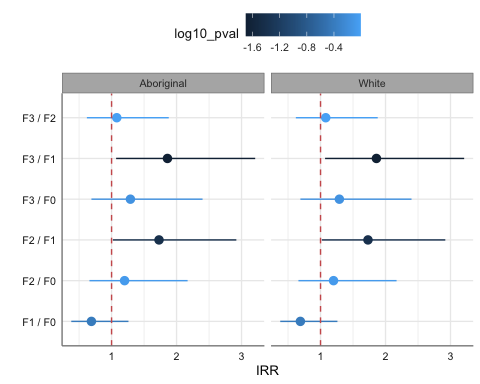# 5 Survival Analysis

We will use an example on the effect of thiotepa versus placebo on the development of bladder cancer.

data(bladder)
Warning in data(bladder): data set 'bladder' not found
bladder <- bladder %>%
mutate(times = stop,
rx = factor(rx, labels=c("Placebo", "Thiotepa"))
) %>%
var_labels(times = "Survival time",
rx = "Treatment")

## 5.1 Parametric method

model_surv <- survreg(Surv(times, event) ~ rx, data = bladder)

Using robust standard errors:

model_surv %>%
glm_coef(labels = c("Treatment: Thiotepa/Placebo", "Scale"), se_rob = TRUE) %>%
as_hux() %>% set_align(everywhere, 2:3, "right") %>%
theme_pubh(1) %>%
add_footnote(get_r2(model_surv), font_size = 9)
ParameterSurvival time ratioPr(>|z|)
Treatment: Thiotepa/Placebo1.64 (0.89, 3.04)0.116
Scale1 (0.85, 1.18)0.992
Nagelkerke's R2 = 0.02

In this example the scale parameter is not statistically different from one, meaning hazard is constant and thus, we can use the exponential distribution:

model_exp <- survreg(Surv(times, event) ~ rx, data = bladder, dist = "exponential")
model_exp %>%
glm_coef(labels = c("Treatment: Thiotepa/Placebo"), se_rob = TRUE) %>%
as_hux() %>% set_align(everywhere, 2:3, "right") %>%
theme_pubh() %>%
add_footnote(get_r2(model_exp), font_size = 9)
ParameterSurvival time ratioPr(>|z|)
Treatment: Thiotepa/Placebo1.64 (0.85, 3.16)0.139
Nagelkerke's R2 = 0.02

Interpretation: Patients receiving Thiotepa live on average 64% more than those in the Placebo group.

Using naive standard errors:

model_exp %>%
glm_coef(labels = c("Treatment: Thiotepa/Placebo")) %>%
as_hux() %>% set_align(everywhere, 2:3, "right") %>%
theme_pubh() %>%
add_footnote(get_r2(model_exp), font_size = 9)
ParameterSurvival time ratioPr(>|z|)
Treatment: Thiotepa/Placebo1.64 (1.11, 2.41)0.012
Nagelkerke's R2 = 0.02
model_exp %>%
plot_model(type = "pred", terms = ~ rx, dot.size = 1.5, title = "") %>%
gf_labs(y = "Survival time", x = "Treatment", title = "")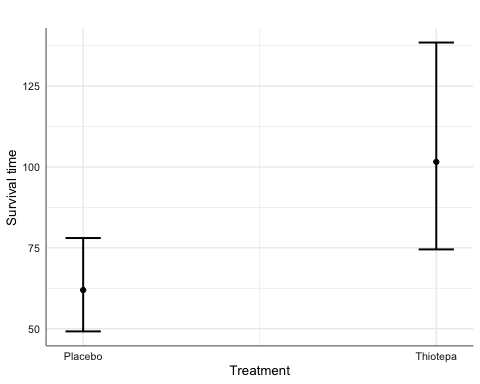## 5.2 Cox proportional hazards regression

model_cox <-  coxph(Surv(times, event) ~ rx, data = bladder)
model_cox %>%
tbl_regression(exponentiate = TRUE) %>%
cosm_reg() %>% theme_pubh() %>%
add_footnote(get_r2(model_cox), font_size = 9)
 Variable HR 95% CI p-value Treatment 0.022 Placebo — — Thiotepa 0.64 0.44, 0.94 Nagelkerke's R2 = 0.016

Interpretation: Patients receiving Thiotepa are 64% less likely of dying than those in the Placebo group.